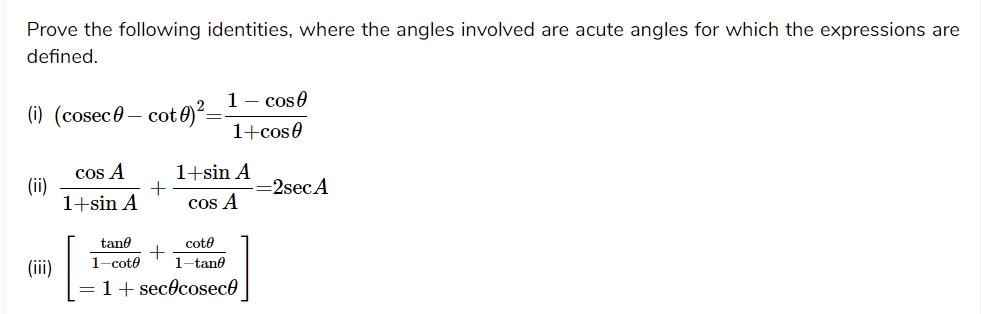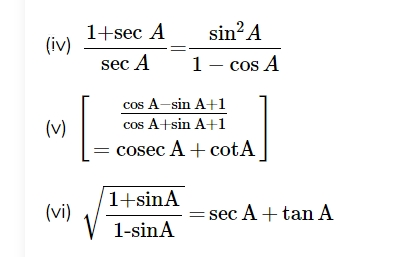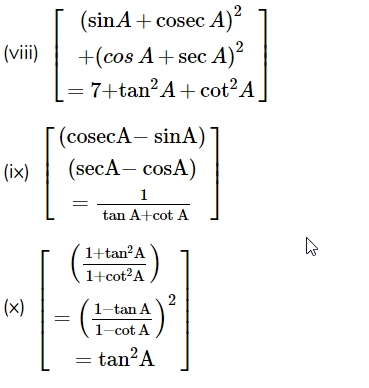NCERT Class 10 Maths Chapter 8 Trigonometry Free video classes and solutionsvictersPosting FreakPosts: 968 Threads: 384 Joined: Jul 2020 11-26-2020, 10:36 AM Welcome to the free live video classes of the chapter 8 Introduction to Trigonometry. The classes are taken by our maths expert Smt Radhika Polina and you will get thorough in all the basic concepts in this introductory class. NCERT Class 10 Maths Video Lessons and exercise solutions Introduction to Trigonometry| Part-1|Explanation of Basic Concepts In this video teacher explains the concepts of Trigonometric Ratios in detail Watch the class in YouTubevictersPosting FreakPosts: 968 Threads: 384 Joined: Jul 2020 11-26-2020, 10:50 AM NCERT Class 10 Maths Video Lessons and exercise solutions Introduction to Trigonometry| Part-2|Explanation of Basic Concepts 2 In this class teacher explains the rest of the topic- trigonometric ratios, trigonometric ratio table, trigonometric identities etc.. Watch the Video class in YouTube Attached FilesTrigonometry Introuction Notes.pdf (Size: 244.5 KB / Downloads: 166)victersPosting FreakPosts: 968 Threads: 384 Joined: Jul 2020 11-26-2020, 11:16 AM NCERT Class 10 Maths Video Lessons and exercise solutions Introduction to Trigonometry| Part-3|Exercise[b] 8.1 Problems (1 to 5) solutionsÂ [/b] 1. InÂ  Î”ABC,Â right-angled at B,Â AB=24cm,,Â BC=7cmcm, determine: (i)Â sinA,cosA (ii)Â sinÂ C, 2. Â In the given figure, findÂ tanPÂ âˆ’cotÂ R. 3. If sin A=3/4Â calculateÂ cosAÂ andÂ tanA. 4. Given 15cotA=8, findÂ sinA andÂ secA. 5. GivenÂ secÎ¸=13/12,Â calculate all other trigonometric ratios. Watch the class in YouTube for the solutionsvictersPosting FreakPosts: 968 Threads: 384 Joined: Jul 2020 11-26-2020, 11:36 AM NCERT Class 10 Maths Video Lessons and exercise solutions Introduction to Trigonometry| Part-4| Exercise 8.1 Problems (6-11) The following problems are taken in this class Â  6.Â IfÂ âˆ A andÂ âˆ B are acute angles such thatÂ cosA=cosB,Â then show thatÂ âˆ A=âˆ B 7. IfÂ cotÎ¸= 7/8 evaluate: (i) (1+sinÎ¸)(1âˆ’sinÎ¸) / (1+cosÎ¸)(1âˆ’cosÎ¸) (ii)Â cot2Î¸ 8. IfÂ 3cotA=4, check whetherÂ  1âˆ’tan2A /1+tan2A =cos2Aâˆ’sin2A or not. 9. In theÂ Î”ABCÂ right-angled atÂ B, ifÂ tanA= 1 /âˆš3Â  find the value of: (i)Â sinÂ AÂ cosÂ C+Â cosÂ AsinÂ C (ii)Â cosÂ A cosÂ Câˆ’Â sinÂ AÂ sinÂ C 10. InÂ Î”PQR,Â right-angled atÂ Q, PR+QR=25cmÂ andÂ PQ=5cm.Â Determine the values ofÂ sinP,cosP andÂ tanP. 11. State whether the following are true or false. Justify your answer. (i) The value ofÂ tanAs always less thanÂ 1. (ii)Â secA= 12/ 5 for some value ofÂ âˆ A. (iii)Â cosA is the abbreviation used for the cosecant ofÂ âˆ A (iv)Â cotA is the product ofÂ cot andÂ A (v)Â sinÎ¸= 4/3 ,Â for someÂ âˆ Î¸ Watch the class in YouTube for the solutionsvictersPosting FreakPosts: 968 Threads: 384 Joined: Jul 2020 11-26-2020, 12:01 PM NCERT Class 10 Maths Video Lessons and exercise solutions Introduction to Trigonometry| Part-5| Exercise 8.2 problems 1 main(i,ii,iii) Teacher explains the following problems in this video (i)Â sin60âˆ˜cos30âˆ˜+sin30âˆ˜cos60âˆ˜ (ii)Â 2tan245âˆ˜+cos230âˆ˜âˆ’sin260âˆ˜ (iii) cos45âˆ˜/ sec30âˆ˜+cosec30âˆ˜ Watch the class in YouTube for the solutionsvictersPosting FreakPosts: 968 Threads: 384 Joined: Jul 2020 11-26-2020, 12:16 PM NCERT Class 10 Maths Video Lessons and exercise solutions Introduction to Trigonometry| Part-6|Problems 1 main(iv,v),2,3&4 Teacher explains the solutions to the following problems (iv)Â  sin30âˆ˜+tan45âˆ˜âˆ’cosec60âˆ˜ /sec30âˆ˜+cos60âˆ˜âˆ’cot45âˆ˜ (v)Â  5cos260âˆ˜+4sec230âˆ˜âˆ’tan245âˆ˜/sec230âˆ˜+cos230âˆ˜ Choose the correct option and justify your choice: (i)Â  2tan30âˆ˜/1+tan230âˆ˜ Â (A)Â sin60âˆ˜ (B)Â cos60âˆ˜ ©Â tan60âˆ˜ (D)Â sin60âˆ˜ (ii)Â  1âˆ’tan245âˆ˜ / 1+tan245âˆ˜ (A)Â tan90âˆ˜ (B)Â 1 ©Â sin45âˆ˜ (D)Â 0âˆ˜ (iii)Â sin2A=2sinA is true whenÂ A= (A)Â 0âˆ˜ (B)Â 30âˆ˜ ©Â 45âˆ˜ (D)Â 60âˆ˜ (iv)Â  2tan30âˆ˜ /1âˆ’tan230âˆ˜ (A)Â cos60âˆ˜ (B)Â sin60âˆ˜ ©Â tan60âˆ˜ (D)Â Â sin30âˆ˜ 3. IfÂ tan(A + B)=âˆš3t and tan(Aâˆ’B)= 1/âˆš3 ;0âˆ˜<(A + B)â‰¤90âˆ˜,A>B, findÂ A B. Watch the class in YouTube for the solutionsvictersPosting FreakPosts: 968 Threads: 384 Joined: Jul 2020 11-26-2020, 12:24 PM NCERT Class 10 Maths Video Lessons and exercise solutions Introduction to Trigonometry| Part-7| Exercise 8.3 Teacher explains the following question in this class 8.3.1 Evaluate: (i)Â Â  sin180/ cos720 (ii)Â  tan260/ cot640 (iii)Â cos480âˆ’sin420 (iv)Â cosec310âˆ’sec590 8.3.2Â  Show that: (i)Â tan48âˆ˜tan23âˆ˜tan42âˆ˜Â tan67âˆ˜=1 (ii)Â cos38âˆ˜cos52âˆ˜âˆ’sin38âˆ˜Â sin52âˆ˜=0 8.3.3Â IfÂ tan2A=cot(Aâˆ’18âˆ˜), whereÂ 2AÂ an acute angle, find the value ofÂ A. 8.3.4Â IfÂ tanA=cotB,Â prove thatÂ A+B=90âˆ˜. 8.3.5Â Â IfÂ sec4A=cosec(Aâˆ’20âˆ˜),,whereÂ 4AÂ is an acute angle, find the value ofÂ A. 8.3.6Â IfÂ A,Â BÂ andÂ CÂ are interior angles of a triangleÂ ABC, then show that sin(B+C/2Â )=cosÂ A/Â 2 8.3.7Â ExpressÂ sin67âˆ˜+cos75âˆ˜Â in terms of trigonometric ratios of angles betweenÂ 0âˆ˜Â andÂ 45âˆ˜Â . Watch the class in YouTube for the solutionsvictersPosting FreakPosts: 968 Threads: 384 Joined: Jul 2020 11-26-2020, 12:41 PM NCERT Class 10 Maths Video Lessons and exercise solutions Introduction to Trigonometry| Part-8| Exercise 8.4 Problems ((1,2,3 & 4) 8.4.1 Express the trigonometric ratiosÂ sinA,Â secA andÂ Â tanA in terms ofÂ cotA. 8.4.2 Write all the other trigonometric ratios ofâˆ A in terms ofÂ secA 8.4.3 Evaluate (i)Â  sin263âˆ˜+sin227âˆ˜ /cos217âˆ˜+cos273âˆ˜ (ii)Â sin25âˆ˜cos65âˆ˜+cos25âˆ˜Â sin65âˆ˜ Watch the class in YouTube for the solutionsvictersPosting FreakPosts: 968 Threads: 384 Joined: Jul 2020 11-26-2020, 12:57 PM NCERT Class 10 Maths Video Lessons and exercise solutions Introduction to Trigonometry| Part-8| Exercise 8.4 Problems 5th main(I to III) Teacher solves the followingÂ  problems in this classWatch the class in YouTube for the solutionsvictersPosting FreakPosts: 968 Threads: 384 Joined: Jul 2020 11-26-2020, 01:03 PM NCERT Class 10 Maths Video Lessons and exercise solutions Introduction to Trigonometry| Part-9| Exercise 8.4 Problems 5th main(IV to VI) Teacher introduces the following problems and finds the solutions Prove the following identities, where the angles involved are acute angles for which the expressions are defined.Watch the class in YouTube for the solutionsvictersPosting FreakPosts: 968 Threads: 384 Joined: Jul 2020 11-26-2020, 01:09 PM NCERT Class 10 Maths Video Lessons and exercise solutions Introduction to Trigonometry| Part-10| Exercise 8.4 Problems 5th main(VII to x) In this class the following problems are solved Prove the following identities, where the angles involved are acute angles for which the expressions are definedWatch the class in YouTube

 Possibly Related Threads… Thread Author Replies Views Last Post NCERT Class 10 Maths Chapter 7 Coordinate Geometry Solutions and Free online classes victers 5 3,160 12-12-2020, 12:40 AM Last Post: victers NCERT Class 10 Maths Chapter 9 Problems Solutions and Free video classes victers 5 3,506 12-08-2020, 06:31 PM Last Post: victers NCERT Class 11 Maths Chapter 10 Constructions Problems Solutions and Free classes victers 4 2,900 11-26-2020, 08:59 AM Last Post: victers NCERT Class 10 Maths Chapter 10 Circles Solutions Video Lessons text_book 5 3,584 11-24-2020, 06:42 PM Last Post: text_book NCERT Class 10 Maths Chapter 5 Arithmetic Progression Solutions Video Lessons text_book 16 6,193 11-23-2020, 09:49 AM Last Post: victers NCERT Class 10 Maths Triangles Chapter Problem Solutions & Video Lessons text_book 19 7,863 11-18-2020, 11:21 AM Last Post: text_book NCERT Class 10 Maths Chapter 15- Problem solutions & explanation videos text_book 4 3,026 10-26-2020, 12:32 AM Last Post: text_book NCERT Class 10 Maths Chapter 3- Problem solutions & explanation videos text_book 16 6,718 10-24-2020, 12:25 AM Last Post: text_book

 Users browsing this thread: 1 Guest(s)